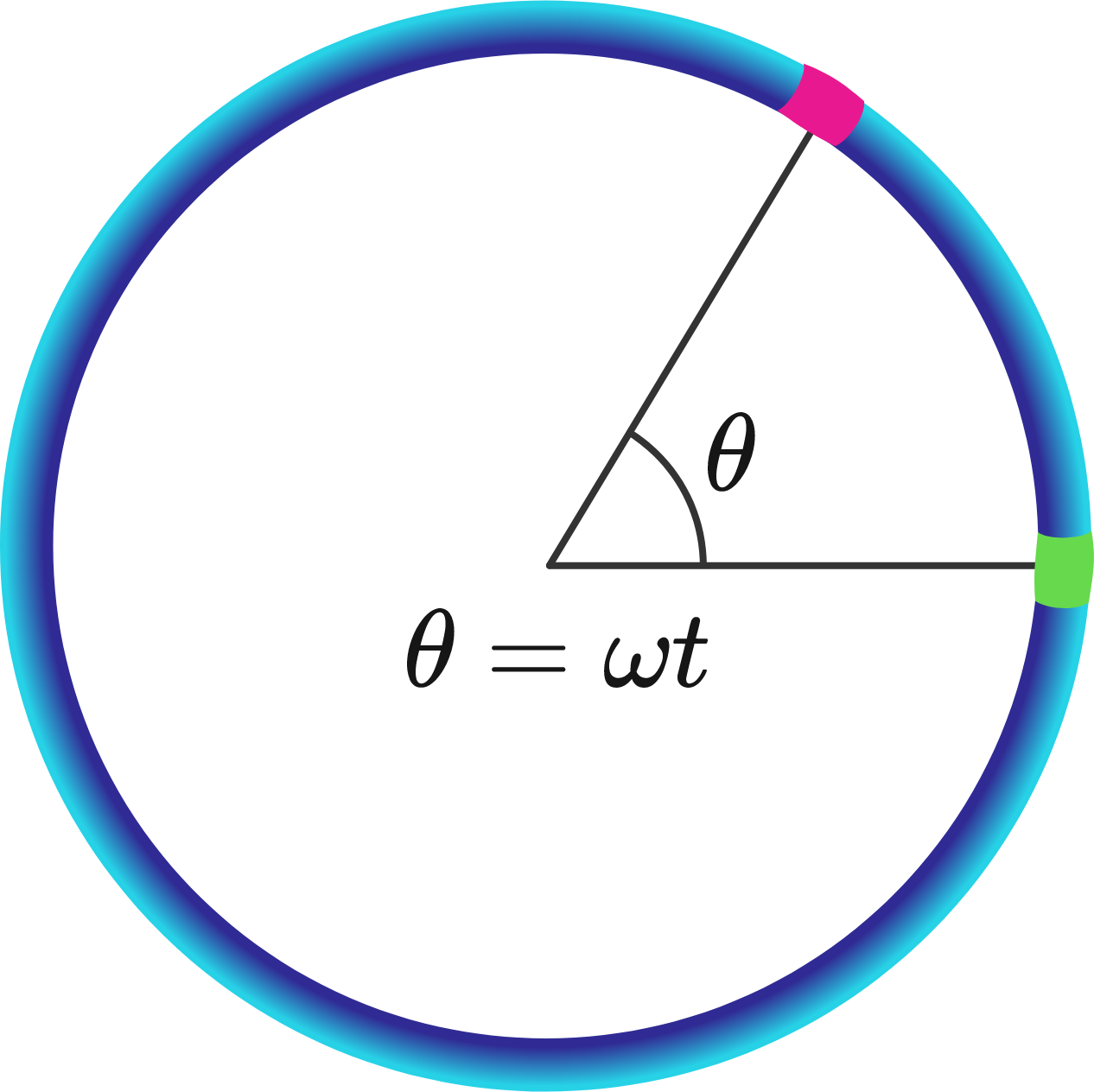# Average Equivalent Ring ResistanceThere are two colored points (purely abstract entities) situated on a conducting circular ring.

The ring has a total electrical resistance $R$, which is uniformly distributed over its circumference.

The green point is fixed at a particular location on the ring (the particular location doesn't matter).

The red point traverses over the ring's circumference at a constant angular speed $\omega$, and its location coincides with that of the green point at time $t = 0$.

The time-averaged (over an integer number of rotational periods beginning at $t = 0$) equivalent resistance between the two points can be expressed as $\large{\frac{R}{\alpha}}$.

Determine the value of $\alpha$.

×